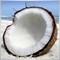MT5 Previous Bar Hi-Lo58

Does anyone happen to know how to convert this to MT5 so I can display the previous bars' high and low:

int counted_bars=IndicatorCounted();

double HIGH;
double LOW;

if((Time!=curTime))

curTime=Time;

HIGH = High;
LOW = Low;

I've tried numerous things but can't seem to find a solution.4171

You can use CopyRates() function to get time, high, low,... values.

This code is written without check in compiler, therefore you probably will need to fix some small errors:

int counted_bars=IndicatorCounted();
double HIGH;
double LOW;
MqlRate barData[];
int copiedAmount = CopyRates(_Symbol, PERIOD_CURRENT, 0, 2, barData);
if (copiedAmount == 2){
ArraySetAsSeries(barData, true);
if((barData.time != curTime)){
curTime = barData.time;
HIGH = barData.high;
LOW = barData.low;
}
}Moderator
189879

Here is the implementation in the indicator:

//+------------------------------------------------------------------+
//|                                               test_indicator.mq5 |
//|                        Copyright 2015, MetaQuotes Software Corp. |
//|                                              http://www.mql5.com |
//+------------------------------------------------------------------+
#property copyright   "2015, MetaQuotes Software Corp."
#property version     "1.00"
#property indicator_chart_window
#property indicator_plots     0

datetime   prevtime=0;
//+------------------------------------------------------------------+
//|                                                                  |
//+------------------------------------------------------------------+
int OnInit()
{
//---
return(INIT_SUCCEEDED);
}
//+------------------------------------------------------------------+
//| Custom indicator iteration function                              |
//+------------------------------------------------------------------+
int OnCalculate(const int rates_total,
const int prev_calculated,
const datetime &time[],
const double &open[],
const double &high[],
const double &low[],
const double &close[],
const long &tick_volume[],
const long &volume[],
{
double HIGH;
double LOW;
if(prevtime==time[rates_total-1])
{
return(rates_total);
}
prevtime=time[rates_total-1];
HIGH= high[rates_total-2];
LOW = low[rates_total-2];
Comment(prevtime,"; ",HIGH,"; ",LOW);
//--- OnCalculate done. Return new prev_calculated.
return(rates_total);
}
//+------------------------------------------------------------------+58

You guys are the best! Thank you so much, I've been struggling with this for days. Thank you.